HOME / Applications / Thermal simulation of Induction heating brazing process

# Thermal simulation of Induction heating brazing process

View 3D results Used Tools:## Introduction

Induction heating process is widely used in a various industrial applications such as welding, forming, brazing, hardening and other non-contact heat treatment processes. It is a fast and an efficient method for heating electrically conducting pieces. Compared to other heating methods, induction heating-based processes have a relatively high heating rate and low energy consumption.

Brazing is a metal-joining process in which two or more metal workpieces are joined together by melting and flowing a filler metal into the joint which has a lower melting point than the metal workpieces. Induction brazing uses the induction heating to melt the filler; in this process the material is placed in a high frequency electromagnetic field produced by an inductor. The use of Induction heating is more efficient than the conventional joining process using gas based heating; this is because it transfers more energy per square meter than an open flame .  The higher rate of energy transfer per square meter results in a faster heating cycle with a guaranteed high safety.

The application of induction-based heating process requires computing the frequency, and the amount of current needed to raise the temperature of the heated metal to a specified degree in a given amount of time.

In this study, we analyze the brazing process based on two copper workpieces held very close to each other to be thermally joined. The joint area is heated to a temperature lower than the melting point of the workpiece material.

We used a Multiphysics FEM analysis to analyze the temperature distribution through a coupled electromagnetic and thermal simulation.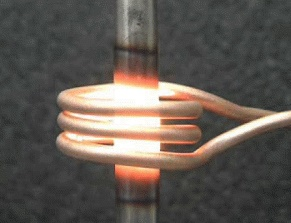Figure 1 - Example of brazing process by induction heating process 

## Multiphysics Analysis in EMS

We used the frequency domain of EMS coupled to the thermal module, and carried out the heat transfer analysis across the metal workpieces within a bounded region. The used 3D model is shown in Figure 2. It consists of a inductor coil with 13 turns surrounding a cylindrical workpiece made up of copper. For the reason of simplification, the workpiece is defined by a one cylindrical bar excluding the filler zone and the results of temperature and current will be calculated across the lines X1 and X2.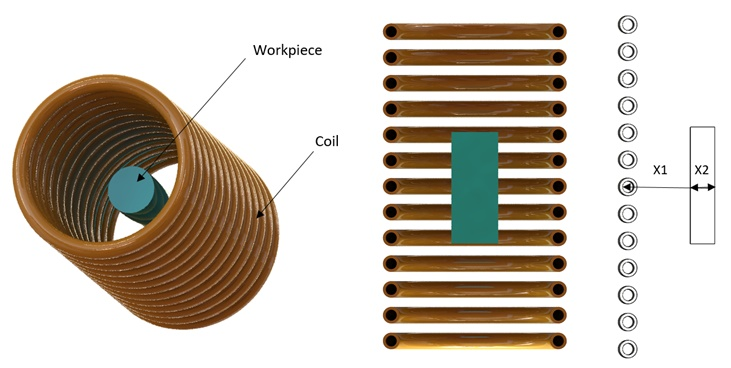Figure 2 - 3D design of the studied model with cross-sectional view

Table 1 - Dimensions of the studied model 
 Part Dimensions (cm) Coil Coil diameter 6 Wire diameter 0.6 Height 11 Number of turns 13 Workpiece Diameter 1.68 Height 4

Both the coil and the workpiece are made with copper material.  The geometrical properties of the workpiece and inductor coil are presented in Table 1. The thermal and electrical properties of the copper used in the Multiphysics simulation are detailed in Table 2.

Table 2 - Material properties
 Material Density (Kg/m²) Magnetic permeability Electrical conductivity (S/m) Thermal conductivity (W/m.K) Specific heat capacity (J/Kg.K) Copper (Cu) 8900 0.99 6 E+07 385 390

## Boundary conditions

### 1- Electromagnetic input:

The copper inductor is defined as a solid coil, supporting a current input of 300A peak value with a frequency of 75 kHz.

### 2- Thermal input:

Thermal convection is applied on the air body surrounding the model at ambient temperature of 22°C with a heat transfer coefficient set to 10 W/ m²C.

## Mesh

The Figure 3 shows the 2D view of the mesh model with a fine mesh control applied to both coil and workpiece to enhance the accuracy of  the  results.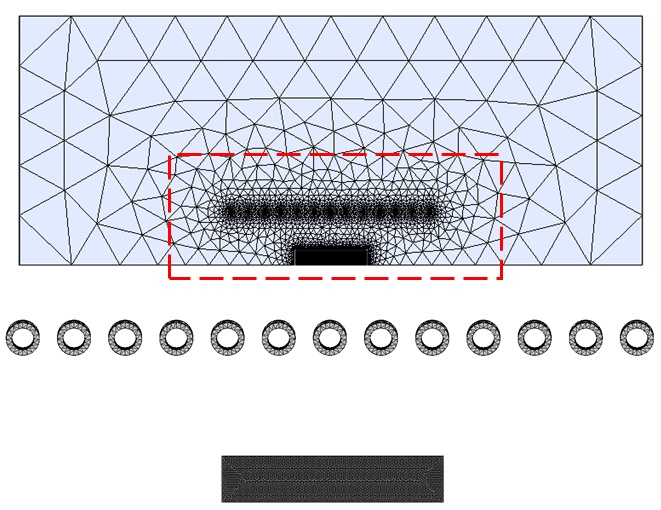Figure 3 - The 2D view of the meshed model

## Results

This section shows the Multiphysics simulation results obtained after one second of heating. The distribution of the magnetic field across the studied model is defined by a 2D cross-sectional plot shown in Figure 4.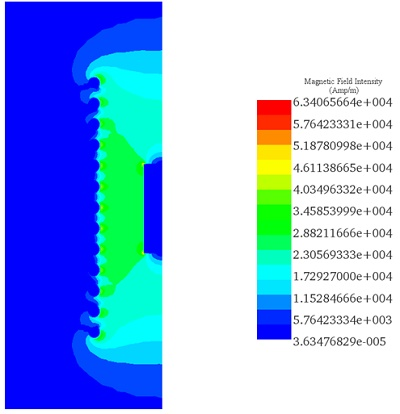Figure 4 - Magnetic field distribution

The Figure 5 presents the 2D plot of the magnetic field intensity across the line X1. As can be seen in Figure 5, the maximum values of magnetic field occur at the area between the external surface of the workpiece and the internal surface of the coil.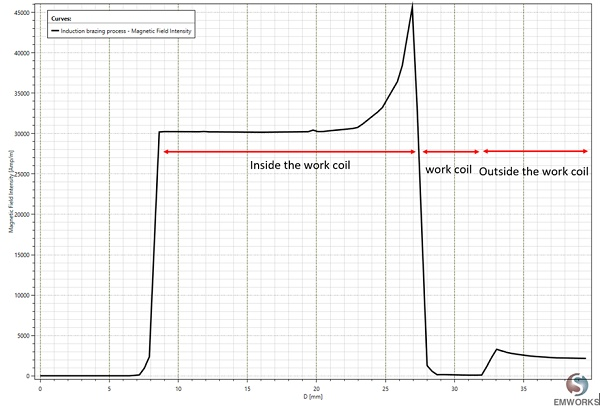Figure 5 - 2D plot of the magnetic field distribution along the line X1

The Figure 6 shows the line plot distribution of the generated magnetic flux which achieves a maximum value of 7.96E-2 Tesla at the edges of the heated workpiece.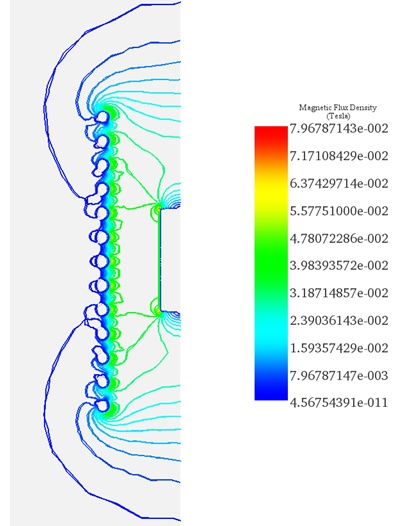Figure 6 - Magnetic flux density distribution

The induced eddy current is localized at the external lateral surface of the copper bar. The figures 7.a) and 7.b) present the current density distribution for an excitation of 300A along the lines X1 and X2 respectively,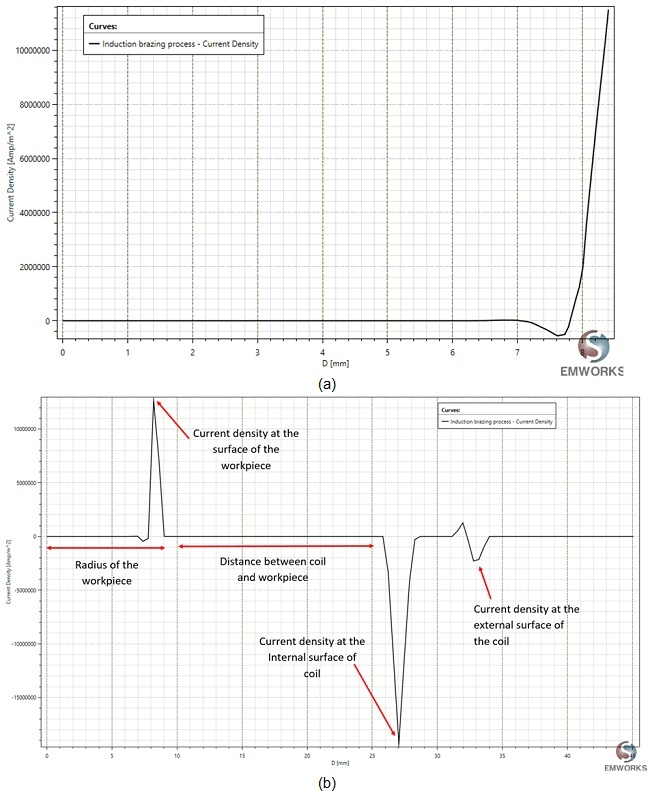Figure 7 -  a). Current density distribution along the line X2 b). X-component of the Current density distribution along the line X1

The ohmic loss results produced by the induced currents are defined through the 2D plot of the Figure 8 along X1 line. Power losses attained a maximum value of 94W at 22°C and at a frequency of 75kHz. These Ohmic losses are transformed to a heat which raises the temperature of the workpiece.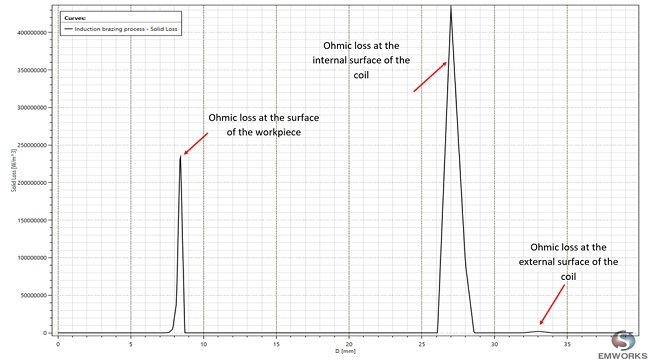Figure 8 - Ohmic losses distribution along X1

On the workpiece, the skin effect causes higher current density on the surface facing the coil.  As the  Ohmic losses continues for one second, the temperature of the workpiece rises from 22 °C maximum to a 28.24 °C as shown in Figure 9. The workpiece temperature is not uniform and fluctuates between 26 °C to 28.2 °C due to non-uniform current distributions.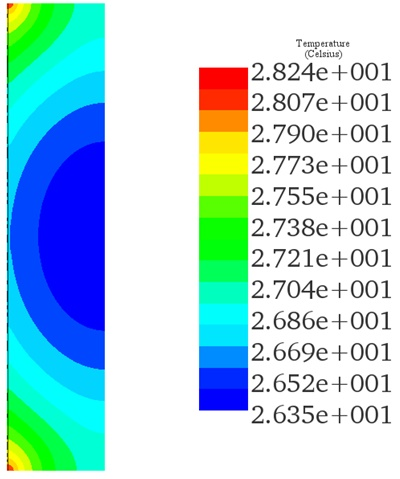Figure 9 - Temperature distribution

## Summary

The induction brazing process provides a fast, clean, accurate and efficient method to heat materials. Before the experimental process, a Multiphysics FEM simulation is required to predict the temperature distribution inside the heated workpiece and to precise the needed current and frequency loading. The FEM-based EMS software serves as a powerful tool to perform induction heating processing through coupling electromagnetic and thermal simulations.

## References

 http://www.efd-induction.com/en/Applications/Brazing.aspx
 https://vacaero.com/information-resources/vacuum-brazing-with-dan-kay/1463-induction-brazing-vs-vacuum-brazing.html
 Khazaal, M. H., & Abdulbaqi, I. M. (2016, May). Modeling, design and analysis of an induction heating coil for brazing process using FEM. In Multidisciplinary in IT and Communication Science and Applications (AIC-MITCSA), Al-Sadeq International Conference on (pp. 1-6). IEEE.

View 3D model and 3D results

Upcoming Webinars